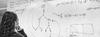# 2nd Semester 2021/22: Transfinite Computability

Instructors
Yurii Khomskii and Lorenzo Galeotti
ECTS
6
Description

Classical computability theory deals with models of computation idealizing real computing, i.e., a process that can be carried out by a physical device, using a finite algorithm and a finite amount of space and time. Transfinite computability refers to various abstractions of this concept from the finite to the infinite.

The earliest such abstraction was the Infinite Time Turing Machine (ITTM) considered by Joel Hamkins and Andy Lewis, 2000, in which a Turing Machine was allowed to run in transfinite time, but the mechanics of the machine itself (tape of length omega, finitely many states, finite algorithm) remained unchanged.

In the years to follow, several other models of transfinite computation have been proposed, changing and generalizing other parameters, such as: length of the tape, infinitely many states, infinitely long algorithms, what happens at limit stages of the computation, automata other than the Turing Machine, etc.

Organisation

In this project, students will study and present a particular topic from this research area, in a seminar format. We will mostly follow the recent book:

• Merlin Carl, "Ordinal Computability, An Introduction to Infinitary Machines", De Gruyter Series in Logic and Its Applications, Volume 9. doi.org/10.1515/9783110496154

Each student will present a section from this book, possibly supplemented by other material such as a research article. In case of a large number of participants, group presentations will be possible.

Each student will write and submit a short report summarizing the material they presented.

Prerequisites
• Basic knowledge of recusion theory (Turing Machines, computable function, decidable and semi-decidable sets, Halting set etc.)
• Basic knowledge of set theory (ordinals and cardinals, ordinal arithmetic, cardinal arithmetic, cofinality, singular and regular cardinals).
Assessment

Student presentations and final write-up

References

Merlin Carl, "Ordinal Computability, An Introduction to Infinitary Machines",  De Gruyter Series in Logic and Its Applications, Volume 9. doi.org/10.1515/9783110496154